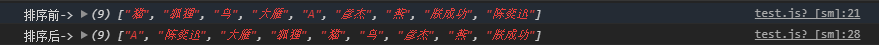# 小程序中英文混合排序问题解决

localeCompare函数排序出来的结果不正确

``` /* 返回拼音的首字母大写 */
getCName(_char) {
if (_char <= 'z' && _char >= 'A') {
return _char.toUpperCase();
}
for (var item in pinyin) {
if (pinyin[item].indexOf(_char) != -1)
return item.toUpperCase();
}
},
/* 对二维数组排序,并返回处理完逻辑的结果 */
sortTwoArr(arr) {
let res_arr=[];
for (var j = 0; j < arr.length; j++) {
for (var i = 0; i < arr.length - 1; i++) {
if (arr[i+1] < arr[i]) {
let temp = arr[i];
arr[i] = arr[i+1];
arr[i+1] = temp;

let temp_ = arr[i];
arr[i] = arr[i+1];
arr[i+1] = temp_;
}
}
}
arr.forEach((item)=>{
res_arr.push(item)
})
return res_arr;
}```

``` /**
* 生命周期函数--监听页面加载
*/
onLoad: function(options) {
let arr = ['猫', '狐狸', '鸟', '大雁', 'A','彦杰','燕','朕成功','陈奕迅'];
let arr1 = [];
arr.forEach((item, index) => {
var _char = item.substr(0, 1);
let temp = [this.getCName(_char), item]
arr1.push(temp);
})
console.log(this.sortTwoArr(arr1))
},```• 相关链接：
• 模板说明：

微信小程序开发-小程序中英文混合排序问题解决